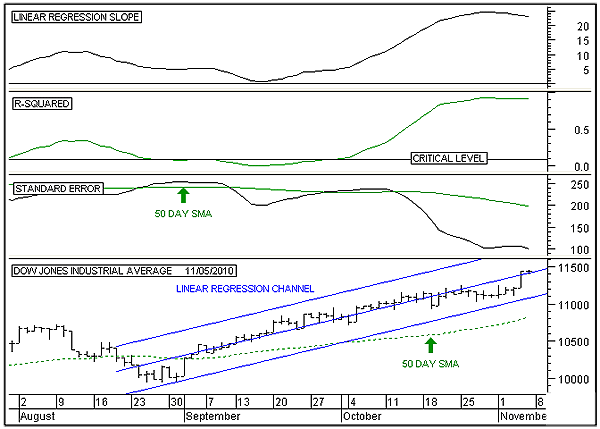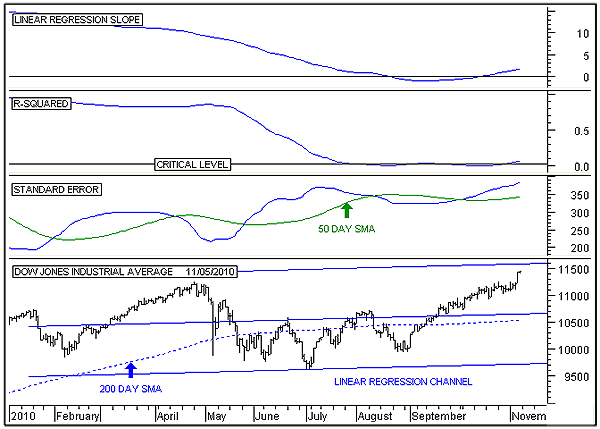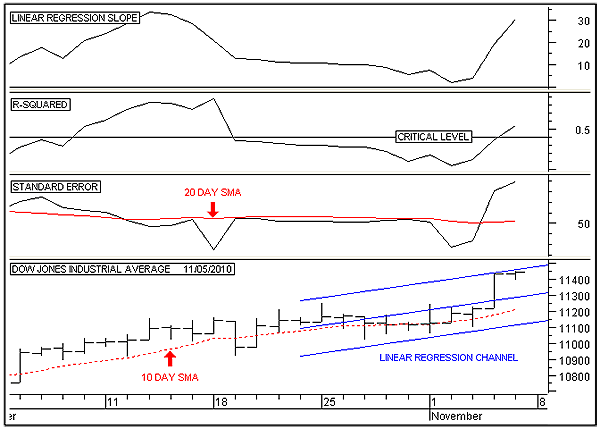HOT TOPICS LIST

INDICATORS LIST

LIST OF TOPICS

# A Linear Regression Analysis Of The DJIA

11/09/10 08:36:29 AM
by Alan R. Northam

Linear regression analysis is used here to show that the Dow Jones Industrial Average is most likely in the very early stage of rolling over to the downside.

Security:   DJI
Position:   N/A

 Figure 1 shows the 50-day linear regression of the Dow Jones Industrial Average (DJIA) and represents the intermediate-term time frame. The bottom panel shows the daily price bars over the last three months. This panel also shows the linear regression channel with the middle line being the linear regression line. The dotted green line is the 50-day simple moving average (SMA) added for reference. The top panel is the linear regression slope indicator and measures the slope of the linear regression line representing the direction of the trend in price. When it crosses above zero and points upward, it indicates that the price trend is accelerating upward. When it moves sideways and is above zero, it indicates that the price trend is no longer accelerating higher. When it is above its zero line and is pointing down, it indicates price deceleration, which normally occurs near the top of a trend. Note that it formed a peak at the end of October 2010 and is now moving downward, indicating that price is starting to decelerate and warns of a change in trend ahead. The next panel is the R-squared indicator and measures the strength of the trend ranging from zero to 1. Currently, it measures 0.9, a very strong trend. Note that it has been moving sideways over the last two weeks, indicating that the strength of the trend is at an extreme. Normally, when a trend is extremely strong, it soon tires and starts to weaken by pointing downward. The third panel down is the standard error indicator, a measure of volatility. This indicator is currently well below its 50-day moving average, indicating low volatility. Low volatility is normally followed by high volatility, and high volatility normally occurs near market tops and bottoms. Once the standard error indicator turns upward and the R-squared indicator turns down, a market top will soon develop. The overall intermediate-term analysis indicates that price is near a top.FIGURE 1: DJIA, DAILY. Here's the DJIA with 50-day linear regression channel. The figure also shows the linear regression slope, R-squared, and standard error indicators. Graphic provided by: MetaStock. Figure 2 is the 200-day linear regression of the DJIA and represents the intermediate-term time frame. The bottom panel shows the daily price bars from the beginning of 2010. This panel shows the 200-day linear regression channel, with the middle line being the linear regression line. The dotted blue line is the 200-day simple moving average added for reference. Note that price is approaching the upper channel line at just above 11500. The top panel is the linear regression slope indicator and measures the slope of the linear regression line, representing the direction of the trend in price. Note that it crossed above its zero line in mid-October, indicating that the long-term trend of price is now upward. Note the shallow nature of the uptrend. When the linear regression slope indicator is at zero, the linear regression channel is horizontal and represents a trading range. However, since the indicator is now starting to point upward, the linear regression channel is starting to slope upward as well. As long as the linear regression slope indicator continues to point higher, the linear regression channel will continue to increase its overall slope. The next panel is the R-squared indicator and measures the strength of the trend. Currently, this indicator has crossed above its critical line. This line is mathematically derived and represents the point at which a new uptrend can be identified with a 95% probability of accuracy. The third panel down is the standard error indicator, a measure of volatility. This indicator is currently above its 50-day moving average and is at its highest level since the beginning of 2010. High volatility normally occurs near market tops. The long-term analysis indicates a weak long-term upsloping trading channel, with high volatility signaling a bounce off the upper linear regression channel line and a return to the middle regression line or even the lower channel line.FIGURE 2: DJIA, DAILY. Here's the DJIA with 200-day linear regression channel. The figure also shows the linear regression slope, R-squared, and standard error indicators. Graphic provided by: MetaStock. Figure 3 is the 10-day linear regression of the DJIA and represents the short-term time frame. The bottom panel shows the daily price bars over the last month. This panel shows the 10-day linear regression channel and middle linear regression line. The dotted red line is the 10-day simple moving average added for reference. Note that price is at its upper channel line. A valid linear regression channel contains all price movement. Normally, price will either continue along this upper channel line, bounce off it, and return to the middle linear regression line, or move down toward the lower channel line where it should again bounce. Eventually, the channel will be broken and a change in trend will develop. The top panel is the linear regression slope indicator and measures the slope of the linear regression line representing the direction of the trend in price. Note that it continues to point upward. As long as this indicator continues to point higher, the linear regression channel will continue to steepen and price will continue to accelerate higher. The next panel is the R-squared indicator and measures the strength of the trend. Currently, this indicator has crossed above its critical line, indicating the presence of a significant trend. Note that the critical line moves up or down based upon the length of the linear regression line. The third panel down is the standard error indicator, a measure of volatility. This indicator is currently well above its 20-day moving average, indicating above average volatility. The short-term analysis indicates higher prices ahead.FIGURE 3: DJIA, DAILY. Here's the DJIA with 10-day linear regression channel. This figure also shows the linear regression slope, R-squared, and standard error indicators. Graphic provided by: MetaStock. In conclusion, the long-term linear regression analysis indicates a very shallow trading channel in progress, indicating either a market top formation or a long-term trading range. Note also that price is now near the upper trading channel line, where a reversal is expected. The intermediate-term linear regression analysis indicates that the uptrend is now starting to decelerate as price approaches the long-term upper channel line. This deceleration is an indication that price is starting the process of rolling over to the downside. The short-term linear regression analysis indicates that even though price is starting to decelerate, it will continue to move upward at a decreasing rate as it moves closer to the long-term channel line before finally turning lower. Overall, the analysis indicates that price is very near a top and a change in trend is soon called for. A breakout above the linear regression channel lines in any of the three time frames will modify this analysis, and a breakdown below any of the linear regression channel lines will confirm it.

Alan R. Northam

Alan Northam lives in the Dallas, Texas area and as an electronic engineer gave him an analytical mind from which he has developed a thorough knowledge of stock market technical analysis. His abilities to analyze the future direction of the stock market has allowed him to successfully trade of his own portfolio over the last 30 years. Mr. Northam is now retired and trading the stock market full time. You can reach him at inquiry@tradersclassroom.com or by visiting his website at http://www.tradersclassroom.com. You can also follow him on Twitter @TradersClassrm.

 Comments or Questions? Article Usefulness 5 (most useful) 4 3 2 1 (least useful)